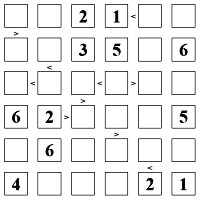Worksheets and No Prep Teaching Resources
Critical Thinking Puzzles
Make Puzzles

# >Greater> <Less< Than Puzzles Puzzle Workbooks for Kids - Updated Each Month!

This page contains Greater Than, Less Than Puzzles. These puzzles are a blend of Sudoku and Magic Squares. Each row contains the numbers 1-5 (or 1-6, 1-7, etc.; customization is always up to you). Students determine where the numbers go based on the numbers already in the puzzle, along with the presence of < and > signs. When the puzzle is completed correctly, the signs and numbers will make correct equations. These puzzles are wonderful for developing logic and critical thinking skills. There are several levels of difficulty available, and the puzzles can use either positive or negative numbers.Easier - >Greater> <Less< Than Puzzles - Some extra numbers given

Easier: Positive Numbers
5 by 5 easy puzzle (2 extra numbers given)
6 by 6 easy puzzle (3 extra numbers given)
7 by 7 easy puzzle (3 extra numbers given)
8 by 8 easy puzzle (4 extra numbers given)
9 by 9 easy puzzle (4 extra numbers given)
10 by 10 easy puzzle (5 extra numbers given)

Easier: Negative Numbers
5 by 5 easy negative number puzzle (2 extra numbers given)
6 by 6 easy negative number puzzle (3 extra numbers given)
7 by 7 easy negative number puzzle (3 extra numbers given)
8 by 8 easy negative number puzzle (4 extra numbers given)
9 by 9 easy negative number puzzle (4 extra numbers given)

>Greater> <Less< Than Puzzles

Positive Numbers
5 by 5 puzzle
6 by 6 puzzle
7 by 7 puzzle
8 by 8 puzzle
9 by 9 puzzle
10 by 10 puzzle

Negative Numbers
5 by 5 negative number puzzle
6 by 6 negative number puzzle
7 by 7 negative number puzzle
8 by 8 negative number puzzle
9 by 9 negative number puzzle

>Greater> <Less< Than Puzzles - Students may use any number from 1 to 9

Positive Numbers - Using all numbers 1 to 9
5 by 5 puzzle (using numbers 1 to 9)
6 by 6 puzzle (using numbers 1 to 9)
7 by 7 puzzle (using numbers 1 to 9)
8 by 8 puzzle (using numbers 1 to 9)

Negative Numbers - Using all numbers -1 to -9
5 by 5 negative number puzzle (using all numbers -1 to -9)
6 by 6 negative number puzzle (using all numbers -1 to -9)
7 by 7 negative number puzzle (using all numbers -1 to -9)
8 by 8 negative number puzzle (using all numbers -1 to -9)

Have a suggestion or would like to leave feedback?
Leave your suggestions or comments about edHelper!